# Prove d2 is a metric d2 : R2 x R2 - R (r, y) max{a 2...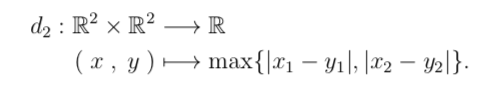Prove d2 is a metric

d2 : R2 x R2 - R (r, y) max{a 2 - y2}.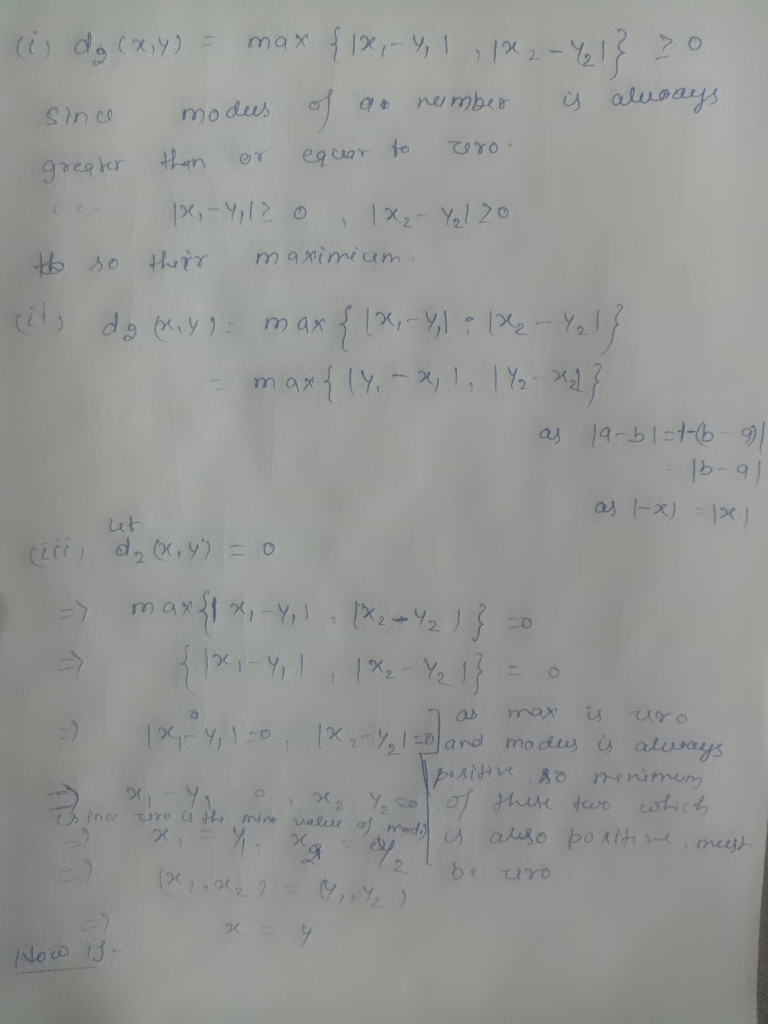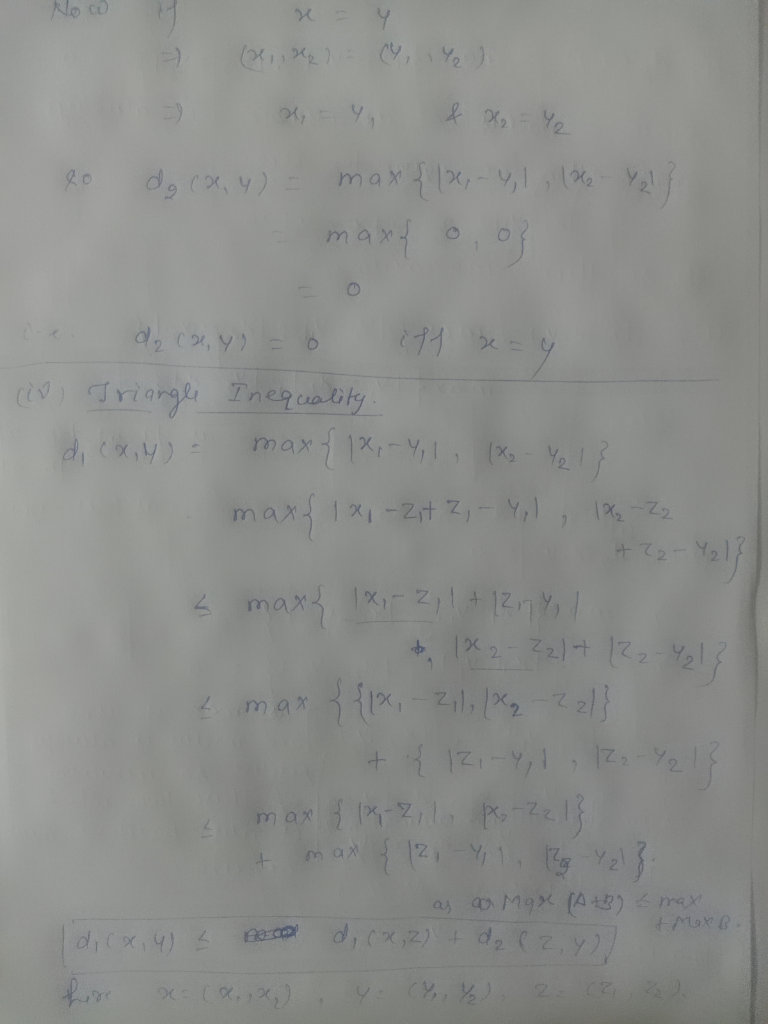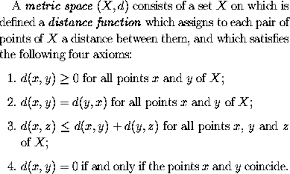##### Add Answer of: Prove d2 is a metric d2 : R2 x R2 - R (r, y) max{a 2...
Similar Homework Help Questions
• ### Metric Spaces help

how to prove that the following is a metric space:a) (E1 x E2, d) where (E1, d1), (E2, d2) are metric spaces and d is given by d( (x1, x2), (y1,y2)) = max {d1 (x1, y1), d2 (x2, y2)}andb) all bounded infinite sequences x = (x1,x2,...) of elements R, withd(x,y) = l.u.b.{|x1-y1|,|x2-y2|,...}

• ### Metric Space

R2=Xd2=R2xR2->R(x1, x2), (y1, y2)->√((y1-x1)2+(y2-x2)2)show that d2 is a metric on R2

• ### Metric Space

R2=Xd2=R2xR2->R(x1, x2), (y1, y2)->√((y1-x1)2+(y2-x2)2)show that d2 is a metric on R2

• ### help me solve 5a, and 5b, topology 5. (20 points) (a) On R2, prove that di (,v), (2a) 1 2l (b) Assume that doo((E1,i), (2, v2)) max 2l, l l is a metric. val is a metric on R2 for each p 2 1. Pro...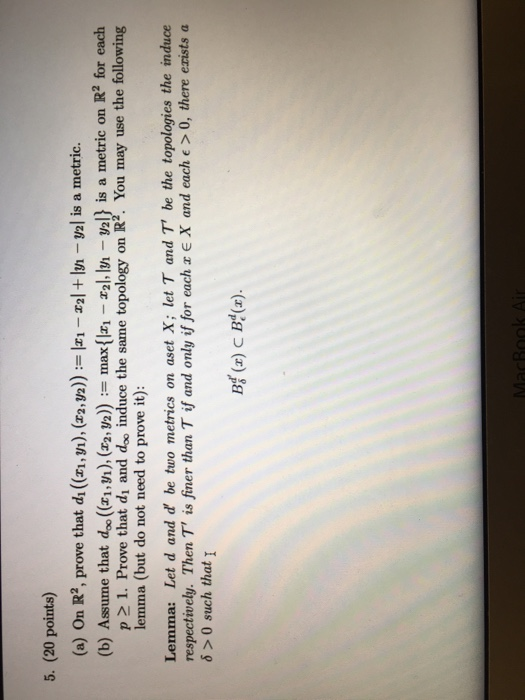help me solve 5a, and 5b, topology 5. (20 points) (a) On R2, prove that di (,v), (2a) 1 2l (b) Assume that doo((E1,i), (2, v2)) max 2l, l l is a metric. val is a metric on R2 for each p 2 1. Prove that di and doo induce the same topology on R2. You may use the following lemma (but do not need to prove it): Lemma: Let d and d be two metrics on aset X; let...

• ### Hi. I'm having trouble with this question in my Topology class. Can I get some help on this?? Tha...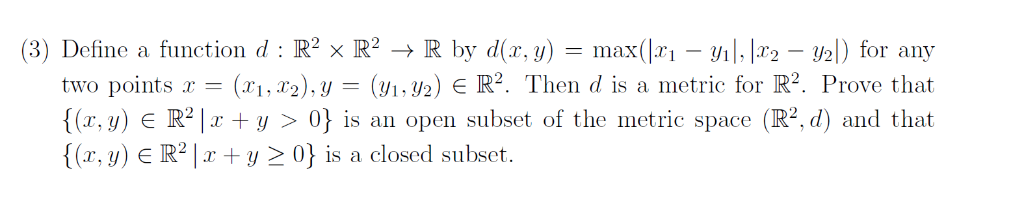Hi. I'm having trouble with this question in my Topology class. Can I get some help on this?? Thank you. (3) Define a function d: R 2 x R2 → R by d(x, y) = max(ki-yil. 12.2-U21) for any two points x-(xi,T2), y-(yi,y2) є R2. Then d is a metric for R2. Prove that {(r,y) є R2lr+y > 0} is an open subset of the metric space (R2, d) and that {(x, y) є R2 1 x + y >...

• ### Problem 2: For any x, y e R let d(x,y):-arctan(y) - arctan(x). Do the following: (1) Prove that d...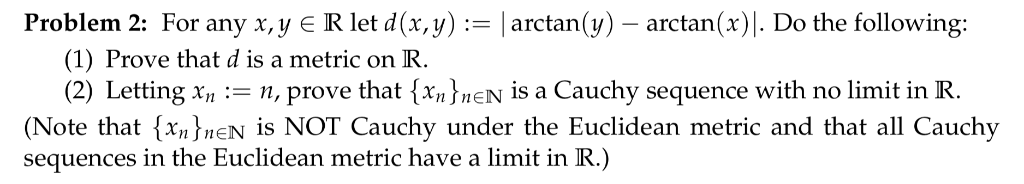Problem 2: For any x, y e R let d(x,y):-arctan(y) - arctan(x). Do the following: (1) Prove that d is a metric on R. (2) Letting xnn, prove that {xnJnE is a Cauchy sequence with no limit in R (Note that {xn)nen is NOT Cauchy under the Euclidean metric and that all Cauchy sequences in the Euclidean metric have a limit in R.) Problem 2: For any x, y e R let d(x,y):-arctan(y) - arctan(x). Do the following: (1) Prove...

• ### {(r, y) E R2 y r} Let A = {A,:r e R} be a collection of sets given by A, = Prove that A is a partition of R2 {(r,...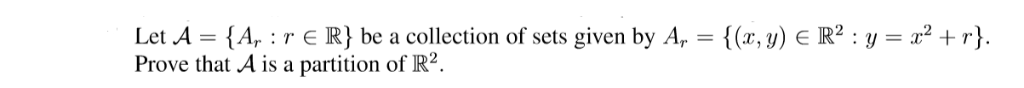{(r, y) E R2 y r} Let A = {A,:r e R} be a collection of sets given by A, = Prove that A is a partition of R2 {(r, y) E R2 y r} Let A = {A,:r e R} be a collection of sets given by A, = Prove that A is a partition of R2

• ### 1(a) Let f : R2 → R b constant M > 0 such that livf(x,y)|| (0.0)-0. Assume that there exists a e continuously differentiable, with Mv/r2 + уг, for all (z. y) E R2 If(x,y)| 〈 M(x2 + y2)· for all (...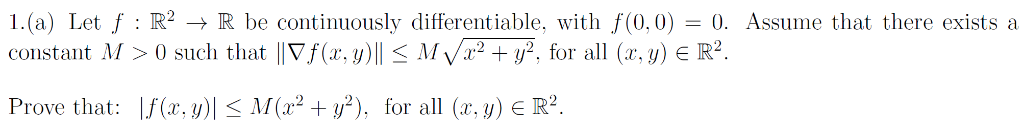1(a) Let f : R2 → R b constant M > 0 such that livf(x,y)|| (0.0)-0. Assume that there exists a e continuously differentiable, with Mv/r2 + уг, for all (z. y) E R2 If(x,y)| 〈 M(x2 + y2)· for all (a·y) E R2 Prove that: 1(a) Let f : R2 → R b constant M > 0 such that livf(x,y)|| (0.0)-0. Assume that there exists a e continuously differentiable, with Mv/r2 + уг, for all (z. y) E R2...

• ### (a) On R2, prove that di ((zı, y), (z2W2)) := Izı-zal + ly,-Val is a metric. (b) Assume that doc ( (zi, yī), (z2,p)) := maxlz-zal, lyi-yl} is a metric on R2 for each p 21. Prove that di and d induce...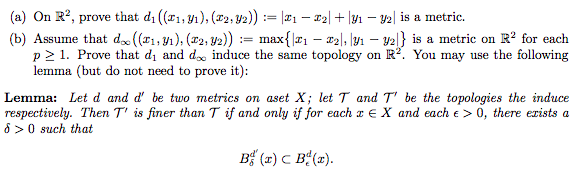(a) On R2, prove that di ((zı, y), (z2W2)) := Izı-zal + ly,-Val is a metric. (b) Assume that doc ( (zi, yī), (z2,p)) := maxlz-zal, lyi-yl} is a metric on R2 for each p 21. Prove that di and d induce the same topology on R2. You may use the following lemma (but do not need to prove it): Lemma: Let d and d' be two metrics on aset X; let T and T' be the topologies the induce...

• ### product of metric spaces

the cartesian product X=X1 times X2 of two metric spaces (X1, d1)and (X2, d2) can be made into a metric space (X, d) in many ways. forinstance, show that a metric space d is defined byd(x,y)= d1(x1,y1) + d2(x2,y2)where x=(x1,x2) and y=(y1,y2)

Free Homework App